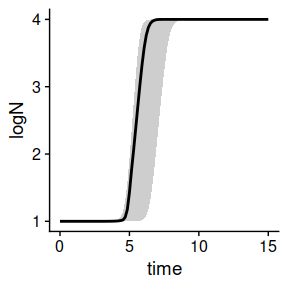# Deprecated and superseded functions

library(biogrowth)
library(tidyverse)

This function describes several functions that have been superseded or deprecated. They are kept here for compatibility with old code that used biogrowth. We recommend to migrate to the new version of the functions (they are linked within the help pages).

# Deterministic modelling

## Growth prediction under static conditions

The biogrowth package includes the function predict_isothermal_growth() to make predictions under static conditions. The calculations are based on primary models (i.e. without secondary models). This function has 3 arguments:

• model_name: a character vector indicating the primary model. Valid values are those returned by primary_model_data.
• times: a numeric vector of storage times to make the predictions.
• model_pars: a named list defining the values of the model parameters.
• check: boolean specifying whether to make some validity checks of model parameters (TRUE by default).

For instance, to make predictions using the modified Gompertz model we would define

my_model <- "modGompertz"

This model has 4 model parameters: mu, lambda, C and logN0 (retrieved using primary_model_data("modGompertz)). All this information must be defined in a list or named vector:

my_pars <- list(logN0 = 2, C = 6, mu = .1, lambda = 50)

Finally, we have to define the storage times for which the prediction is calculated. For instance, we can define 1000 points uniformly distributed between 0 and 200.

my_time <- seq(0, 200, length = 1000)

Once we have defined the arguments, we can call the function predict_isothermal_growth() to get the model predictions. This function makes several checks of the validity of the model parameters before doing the calculations (they can be turned of passing check = FALSE).

static_prediction <- predict_isothermal_growth(my_model, my_time, my_pars)

This function returns an instance of IsothermalGrowth with the results of the simulation. It has three items:

• simulation: A tibble with the results of the simulation.
• model: The name of the model used for making the calculations.
• pars: Vector of model parameters used for the calculations.

We can retrieve the results of the simulation from the simulation item

static_prediction$simulation #> # A tibble: 1,000 × 2 #> time logN #> <dbl> <dbl> #> 1 0 2.00 #> 2 0.200 2.00 #> 3 0.400 2.00 #> 4 0.601 2.00 #> 5 0.801 2.00 #> 6 1.00 2.00 #> 7 1.20 2.00 #> 8 1.40 2.00 #> 9 1.60 2.00 #> 10 1.80 2.00 #> # … with 990 more rows In order to ease interpretation, biogrowth includes a plot S3 method for this class. plot(static_prediction)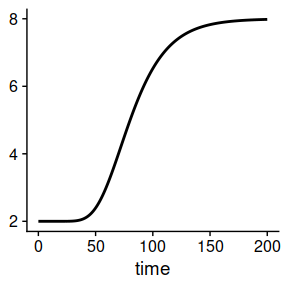The function uses gpplot, so it can be edited using layers as usual in the ggplot2 package. For instance, plot(static_prediction) + xlab("Storage time (h)") + ylab("Microbial count (log CFU/ml)") + theme_gray()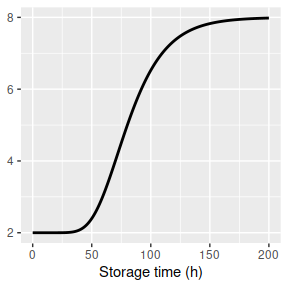The function includes additional arguments to edit the aesthetics of the plot. Please check the hepl page of plot.IsothermalGrowth for a full list of arguments. plot(static_prediction, line_col = "darkgreen", line_size = 2, line_type = 3) + xlab("Storage time (h)") + ylab("Microbial count (log CFU/ml)")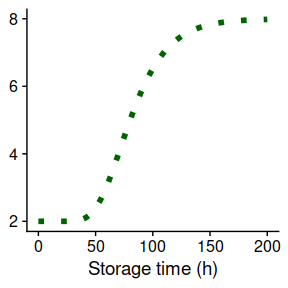## Growth prediction under dynamic conditions The biogrowth package can also be used for simulating growth under dynamic environmental conditions using the predict_dynamic_growth() function. For this, this function combines primary and secondary growth models. It has 7 arguments: • times: Numeric vector of time points for the calculations. • env_conditions: A tibble describing the variation of the environmental conditions. • primary_pars: A named list describing the model parameters of the primary model. • secondary_models: A nested list defining the secondary model(s). • ...: Additional arguments passed to the numeric solver of the differential equation. • check: Whether to do some validity checks of model parameters (TRUE by default). • formula: A one-sided formula describing the x variable in env_conditions. The dynamic environmental conditions are defined using a tibble. It must have a defining the elapsed time and as many additional columns as needed for each environmental factor. By default, the column defining the time must be called time, although this can be changed using the formula argument. For the simulations, the value of the environmental conditions at time points not included in env_conditions is calculated by linear interpolation. In our simulation we will consider two environmental factors: temperature and pH. We can define their variation using this tibble. To illustrate the use of the formula argument, we will use Time for the column describing the elapsed time. my_conditions <- tibble(Time = c(0, 5, 40), temperature = c(20, 30, 35), pH = c(7, 6.5, 5) ) Then, the simulations would consider this temperature profile ggplot(my_conditions) + geom_line(aes(x = Time, y = temperature))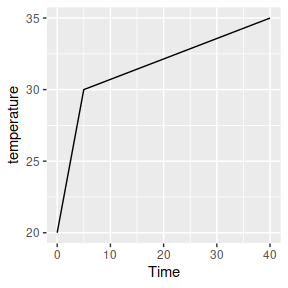And this pH profile ggplot(my_conditions) + geom_line(aes(x = Time, y = pH))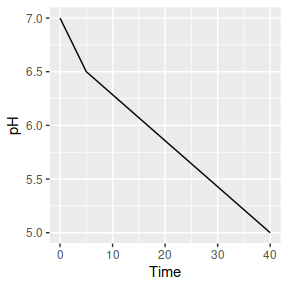We could define smoother profiles using additional rows. For time points outside of the range defined in env_conditions, the value at the closes extreme is used (rule=2 from approx function). For dynamic conditions, biogrowth uses the Baranyi growth model as primary model. This model requires the definition of two model parameters: the specific growth rate at optimum conditions (mu_opt) and the maximum population size (Nmax). Moreover, the initial values of the population size (N0) and the theoretical substance $$Q$$ (Q0) must be defined. Note that $$Q_0$$ is related to the duration of the lag phase under isothermal conditions by the identity $$\lambda = \ln \left( 1 + 1/q_0 \right)/\mu_{max}$$. For the predict_dynamic_growth()function, they all must be defined in a single list: my_primary <- list(mu_opt = .9, Nmax = 1e8, N0 = 1e0, Q0 = 1e-3) The next step is the definition of the secondary models. As already described above, biogrowth describes the variation of $$\mu$$ with temperature based on the gamma concept. Therefore, we need to define one secondary model per environmental condition. This must be done using a list. We define a list per environmental condition that defines the type of gamma model as well as the model parameters. The function secondary_model_data() can aid in the definition of the secondary models. For instance, we will define a gamma-type model for temperature as defined by Zwietering et al. (1992). This is done by including an item called model in the list and assigning it the value "Zwietering". Then, we define the values of the model parameters. In this case, we need the minimum (xmin) and optimum (xopt) cardinal values, as well as the order of the model (n) (this information can be retrieved using secondary_model_data). We define them using individual entries in the list: sec_temperature <- list(model = "Zwietering", xmin = 25, xopt = 35, n = 1) Next, we will define a CPM model for the effect of pH. Note that the model selection is for illustration purposes, not based on any scientific knowledge. First of all, we need to set the item model to "CPM". Then, we need to define the model parameters (note this model also needs xmax). sec_pH <- list(model = "CPM", xmin = 5.5, xopt = 6.5, xmax = 7.5, n = 2) The final step for the definition of the gamma-type secondary model is gathering all the individual models together in a single list and assigning them to environmental factors. Each element on the list must be named using the same column names as in env_conditions. Before, we had used the column names temperature and pH. Thus my_secondary <- list( temperature = sec_temperature, pH = sec_pH ) The final argument is the time points where to make the calculations. We can use a numeric vector with 1000 points between 0 and 50 for this: my_times <- seq(0, 50, length = 1000) Once we have defined every argument, we can call the predict_dynamic_growth() function. Because we are using Time to define the elapsed time in env_conditions, we must also define the .~Time in the formula argument. dynamic_prediction <- predict_dynamic_growth(my_times, my_conditions, my_primary, my_secondary, formula = . ~ Time) This function returns a list of class DynamicGrowth with several items: • simulation: A tibble with the results of the simulation. • gammas: A tibble describing the variation of each gamma factor through the simulation. • env_conditions: Environmental conditions used for the simulations. • primary_pars: Primary model parameters used for the simulations. • sec_models: Secondary model parameters used for the simulations. The results of the simulation can be retrieved from the simulation item: dynamic_prediction$simulation
#> # A tibble: 1,000 × 4
#>      time     Q     N  logN
#>     <dbl> <dbl> <dbl> <dbl>
#>  1 0      0.001     1     0
#>  2 0.0501 0.001     1     0
#>  3 0.100  0.001     1     0
#>  4 0.150  0.001     1     0
#>  5 0.200  0.001     1     0
#>  6 0.250  0.001     1     0
#>  7 0.300  0.001     1     0
#>  8 0.350  0.001     1     0
#>  9 0.400  0.001     1     0
#> 10 0.450  0.001     1     0
#> # … with 990 more rows

We can also visualize the simulation using the S3 method for plot:

plot(dynamic_prediction)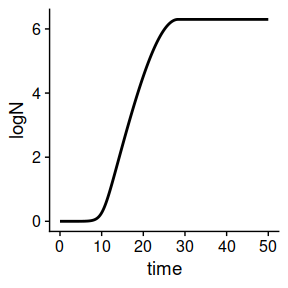The argument add_factor of the plot method can be used to plot the variation of a single environmental factor through storage. For that, one has to pass the name of the desired factor to the function. Note that this name must be identical to the one used for the columns in env_conditions. For instance, we can add the plot of temperature

plot(dynamic_prediction, add_factor = "temperature")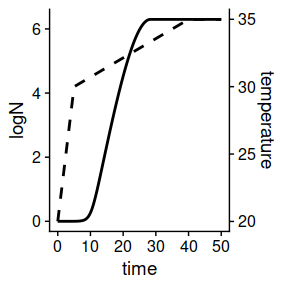The function includes several arguments to edit the aesthetics of the plot. A list of every argument can be found in the help page of plot.DynamicGrowth. The function returns a ggplot object, so it can be further edited using layers.

plot(dynamic_prediction,
ylims= c(0, 7),
label_y1 = "Microbial count (log CFU/ml)",
label_y2 = "Storage temperature (ºC)",
line_col = "lightgreen",
line_size = 2, line_type2 = 1
) +
xlab("Storage time (h)")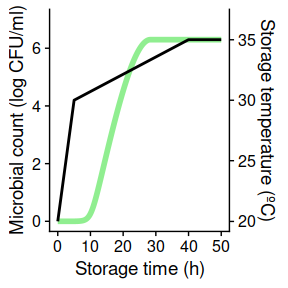## Time to reach a given population size

It is usually of interest to calculate the time required for the population to reach a given size. The biogrowth package includes the function time_to_logcount() for this purpose. This function has 2 arguments:

• model: A model returned by predict_dynamic_growth() or predict_isothermal_growth().
• log_count: target population size.

For instance, we can use this function to estimate the time required to reach a population size of 2.5 log CFU/ml for the static prediction we did earlier:

time_to_logcount(static_prediction, 2.5)
#>  51.98105

Or the time required to reach 5 log CFU/ml in the dynamic prediction:

time_to_logcount(dynamic_prediction, 5)
#>  21.33296

If the value of log_count was outside the range of the simulations, time_to_logcount returns NA:

time_to_logcount(dynamic_prediction, 10)
#>  NA

Note that the calculations are based on linear interpolation of the simulated growth curve. Therefore, its accuracy is strongly dependent on the number of time points used for the simulation. It is recommended to plot the growth curve before doing this calculation. If the curve is not smooth in the area close to the target population size, the simulation should be repeated increasing the number of time points.

## Fitting of primary models

The function fit_isothermal_growth() can be used to estimate the parameters of primary growth models from data obtained under static conditions. This function has 7 arguments:

• fit_data defines the data used for model fitting.
• model_name defines the primary model to use (according to primary_model_data()).
• starting_point defines the initial values of the model parameters (according to primary_model_data()).
• known_pars defines parameters that are considered known and are not fitted to the data.
• ... can be used to pass additional arguments to the modFit() function from the FME package (e.g. lower and upper bounds for the model parameters).
• check states whether to make some validity checks of the model parameters.
• formula defines the names of the x and y variables of the primary model.

The data used for model fitting is defined using the fit_data argument. It must be a tibble with one column defining the elapsed time (time by default), and another one defining the decimal logarithm of the population size (logN by default). For instance, we can use the following tibble, where the elapsed time uses the default column name (time) and the logarithm of the population size uses the name log_size.

my_data <- tibble(time = c(0, 25, 50, 75, 100),
log_size = c(2, 2.5, 7, 8, 8))

In case non-default names are used, they must be defined using the formula argument. The left handside of the equation defines the y-variable, and the right handside the x-variable of the primary model. For the tibble my_data, it would be defined as

my_formula <- log_size ~ time

The primary model is defined using model_name. For instance, we will use the Baranyi model in this example:

my_model <- "Baranyi"

The fit_isothermal_growth() function uses non-linear regression (through the modFit() function of the FME package), so it requires initial values for every model parameter to fit. In the case of the Baranyi model, the model parameters are: logN0, mu, lambda and logNmax (retrieved using primary_model_data("Baranyi")). The fit_isothermal_growth() function enables to fix any model parameter before model fit using the known_pars argument. This can be of interest, as growth models usually have issues related to parameter identifiability. For instance, we can fix the specific growth rate to 0.2 (no particular reason for this, just as a demonstration)

known <- c(mu = .2)

And fit the remaining model parameters

start = c(logNmax = 8, lambda = 25, logN0 = 2)

Once every model parameter has been defined, we can call the fit_isothermal_growth() function. The fitting is done based on the residuals of the logarithm of the population size.

static_fit <- fit_isothermal_growth(my_data, my_model,
start, known,
formula = my_formula
)

This function returns a list of class FitIsoGrowth with several items:

• data: data used for model fitting
• model: name of the primary model
• starting_point: starting point used for model fitting
• known: fixed model parameters
• fit: object returned by modFit()
• best_prediction: an instance of IsothermalGrowth corresponding to the fitted model.

The FitIsoGrowth class includes several S3 methods to help analyzing the results. The statistical information of the fit can be retrieved using summary()

summary(static_fit)
#>
#> Parameters:
#>         Estimate Std. Error t value Pr(>|t|)
#> logNmax  7.99600    0.06980  114.56 7.62e-05 ***
#> lambda  24.64154    0.74220   33.20 0.000906 ***
#> logN0    2.05084    0.09201   22.29 0.002007 **
#> ---
#> Signif. codes:  0 '***' 0.001 '**' 0.01 '*' 0.05 '.' 0.1 ' ' 1
#>
#> Residual standard error: 0.09879 on 2 degrees of freedom
#>
#> Parameter correlation:
#>         logNmax  lambda   logN0
#> logNmax 1.00000 0.05788 0.01589
#> lambda  0.05788 1.00000 0.76437
#> logN0   0.01589 0.76437 1.00000

Besides summary, it also includes methods for residuals, coef, vcov, deviance, fitted and predict to analize the model.

It also includes a plot() method to visually compare the data and the fitted curve

plot(static_fit)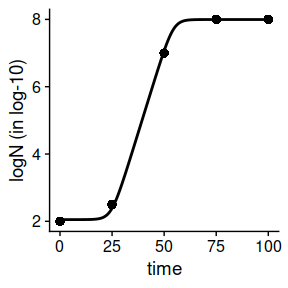The method accepts additional arguments to edit the aesthetics of the plot. A complete list of arguments can be found in the help page of plot. This plot can be edited passing additional arguments toplot.FitIsoGrowth.

plot(static_fit,
line_size = 2, point_col = "lightblue", point_size = 5)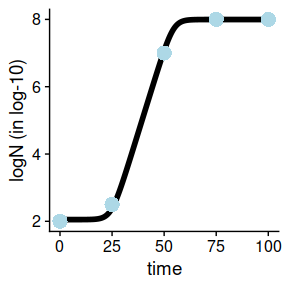Note this method returns an object of class ggplot. Hence, it can be edited using additional layers.

## One-step fitting under dynamic conditions

The function fit_dynamic_growth() can be used to estimate the parameters of both the primary and the secondary model based on a growth experiment obtained under dynamic conditions. This function has 8 arguments:

• fit_data: data used for model fitting.
• env_conditions: variation of the environmental conditions during the experiment.
• starting_point: initial value of the model parameters.
• known_pars: known model parameters (i.e. not fitted).
• sec_model_names: type of secondary model for each environmental factor.
• ...: additional arguments passed to modFit.
• check: whether to do some validity checks of the model parameters (TRUE by default).
• formula: a formula defining the x and y variables of the primary model.

The arguments env_conditions and fit_data are tibbles that describe, respectively, the experimental design and the observations. The biogrowth package includes two example datasets to illustrate the input requirements for this function.

data("example_dynamic_growth")
data("example_env_conditions")

The tibble passed to the argument env_conditions must have a column defining the elapsed time (time by default) and as many additional columns as environmental factors. The fit_dynamic_growth() function is totally flexible with respect to the number of factors or the way they are named. The only requirement is that the definition of every argument is consistent. In the case of example_env_conditions, this dataset considers two factors: temperature and water activity (aw).

head(example_env_conditions)
#> # A tibble: 3 × 3
#>    time temperature    aw
#>   <dbl>       <dbl> <dbl>
#> 1     0          20  0.99
#> 2     5          30  0.95
#> 3    15          35  0.9

Note that for the calculations this function joins the data points by linear interpolation, as shown in this plot:

ggplot(example_env_conditions, aes(x = time, y = temperature)) +
geom_line() +
geom_point()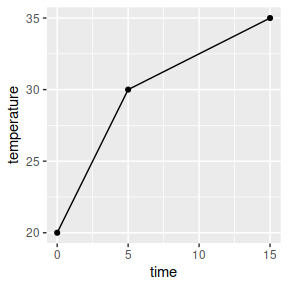The tibble passed to the argument fit_data must have one column defining the elapsed time (time by default) and one defining the logarithm of the population size (logN by default). Different column names can be defined using the formula argument. For instance, formula=log_size ~ Time would mean that the elapsed time is called “Time” and the logarithm of the population size is called “log_size”. Note that the name of the column describing the elapsed time in fit_data must be identical to the one in env_conditions.

head(example_dynamic_growth)
#> # A tibble: 6 × 2
#>    time     logN
#>   <dbl>    <dbl>
#> 1 0      0.0670
#> 2 0.517 -0.00463
#> 3 1.03  -0.0980
#> 4 1.55  -0.0986
#> 5 2.07   0.111
#> 6 2.59  -0.0465

The type of secondary model for each environmental factor is defined by the argument sec_model_names. This argument is a named vector where the names refer to the environmental factor and the value to the type of model. Supported models can be retrieved using secondary_model_data(). In this example we will use cardinal models for both environmental factors. Note that the names of this vector must be identical to the columns in env_conditions.

sec_model_names <- c(temperature = "CPM",
aw= "CPM")

As already mentioned, growth models usually have to deal with parameter identifiability issues. For that reason, the fit_dynamic_growth() function enables to fit or fix any model parameter. This distinction is made using the arguments known_pars (fixed parameters) and starting_point (fitted parameters). Note that every parameter of the primary and secondary model must be in either of these arguments without duplication.

This function uses the Baranyi primary model. It has two variables that need initial values (N0 and Q0) and one primary model parameter (Nmax). The specific growth rate is described using the gamma concept. This requires the definition of its value under optimal conditions (mu_opt) as well as the cardinal parameters for each environmental factor. They must be defined as factor+_+parameter name. For instance, the minimum water activity for growth must be written as aw_xmin.

In this example we will consider the model parameters of the primary model as known. For the secondary model for water activity, we will only consider the optimum value for growth as unknown. Finally, for the effect of temperature, we will consider the order and xmax are known:

known_pars <- list(Nmax = 1e4,  # Nmax for primary model
N0 = 1e0, Q0 = 1e-3,  # Initial values of the primary model
mu_opt = 4, # mu_opt of the gamma model
temperature_n = 1, temperature_xmax = 40,  # Secondary model for temperature
aw_xmax = 1, aw_xmin = .9, aw_n = 1  # Secondary model for water activity
)

Then, the remaining model parameters must be defined in starting_points. Due to the use of non-linear regression for model fitting, it is required to define initial values for these parameters. They can be defined based on previous experience or preliminary numerical simulations.

my_start <- list(temperature_xmin = 25, temperature_xopt = 35,
aw_xopt = .95)

Once every model parameter has been defined, we can call the fit_dynamic_growth() function.

my_dyna_fit <- fit_dynamic_growth(example_dynamic_growth, example_env_conditions,
my_start,
known_pars,
sec_model_names)

The function does some checks of the validity of the model parameters (can be turned off using check=FALSE), raising errors if the model definition does not follow the requirements of the functions. If the fitting was successful, it returns an instance of FitDynamicGrowth with 7 items:

• fit_results: object returned by modFit().
• best_prediction: an instance of DynamicGrowth with the prediction corresponding to the fitted model.
• data: data used to fit the model.
• env_conditions: data used to describe the environmental conditions.
• starting: starting values used for parameter estimation.
• known: model parameters considered known.
• sec_models: type of secondary model for each environmental factor.

FitDynamicGrowth includes an S3 method for summary that returns the statistical information of the fit.

summary(my_dyna_fit)
#>
#> Parameters:
#>                  Estimate Std. Error t value Pr(>|t|)
#> temperature_xmin 26.43224    3.46652   7.625 3.35e-08 ***
#> temperature_xopt 33.85365   10.89999   3.106  0.00443 **
#> aw_xopt           0.99147    0.05574  17.786  < 2e-16 ***
#> ---
#> Signif. codes:  0 '***' 0.001 '**' 0.01 '*' 0.05 '.' 0.1 ' ' 1
#>
#> Residual standard error: 0.1478 on 27 degrees of freedom
#>
#> Parameter correlation:
#>                  temperature_xmin temperature_xopt aw_xopt
#> temperature_xmin           1.0000          -0.9897  0.9762
#> temperature_xopt          -0.9897           1.0000 -0.9971
#> aw_xopt                    0.9762          -0.9971  1.0000

Besides summary, it also includes methods for residuals, coef, vcov, deviance, fitted and predict to analyze the fitted model. Moreover, it includes a plo method to compare the model predictions against the data used for the fit:

plot(my_dyna_fit)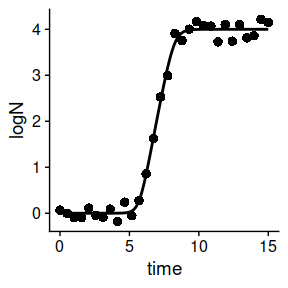The variation of the environmental factor can be plotted alongside the previous plot. For that, the name of the environmental factor must be passed to add_factor. Note that the value passed must be identical to the one defined in the previous arguments. The function provides additional arguments to edit the aesthetics of the plot (a full list can be retrieved from the help page of plot.FitDynamicGrowth). The function returns an instance of ggplot, so it can be edited using additional layers.

plot(my_dyna_fit, add_factor = "aw",
label_y1 = "Log count (log CFU/ml)",
label_y2 = "Water activity",
line_col = "pink",
line_col2 = "yellow",
point_col = "lightgreen") +
theme_dark()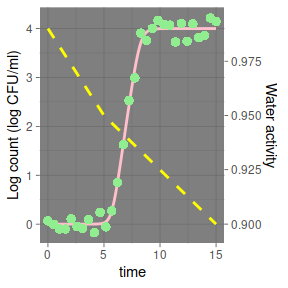## Global fitting of various (dynamic) experiments

The function fit_multiple_growth() can be used to fit one growth model to data gathered in various experiments performed under dynamic conditions. It has several arguments:

• starting_point starting values of the model parameters to fit.
• experiment_data a nested list describing the experimental data and environmental conditions.
• known_pars vector of parameters that are fixed (i.e. not fitted).
• sec_model_names definition of the secondary models for each condition.
• ... additional arguments passed to FME::modFit.
• check whether to do validity checks of the model parameters (TRUE by default).
• formula defines the x and y variables of the primary model.

The data to use for the fit is described using the experiment_data argument. It is a nested list with as many elements as experiments. The dataset multiple_experiments serves as a convenient example for this function.

data("multiple_experiments")

Each experiment is described using a list with two elements: data and conditions. The element data describe the observed variation in the population size using the same convention as fit_data in fit_dynamic_growth().

ggplot(multiple_experiments[]$data) + geom_point(aes(x = time, y = logN))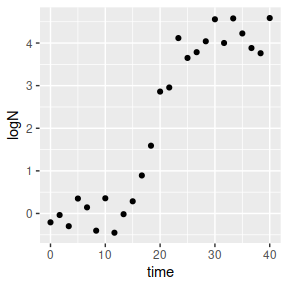The element conditions describes the (dynamic) environmental conditions during storage. It follows the same requirements as env_conditions in fit_dynamic_growth(). Although the function is flexible regarding the number of environmental factors or the column names, they must be consistent for every element in the list. As already mentioned, for every simulation, the values of environmental conditions at times not included in the data frame are calculated by linear interpolation, as illustrated in the next plot.  multiple_experiments[]$conditions %>%
pivot_longer(-time, names_to = "condition", values_to = "value") %>%
ggplot() +
geom_line(aes(x = time, y = value)) +
facet_wrap("condition", scales = "free")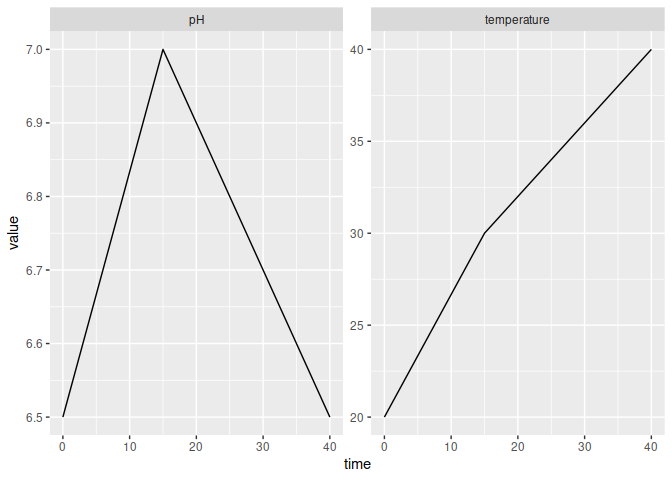The secondary models are defined using sec_model_names, following the same convention as in predict_dynamic_growth(). This argument is a named vector whose names are identical to those in the experimental data and whose values corresponds to valid identifiers according to seccondary_model_data(). For this example, we will use a CPM model for both pH and temperature (for no particular scientific reason).

sec_names <- c(temperature = "CPM", pH = "CPM")

The next step is the definition of model parameters. This is done using the starting_point (for parameters to estimate) and known_pars (for known parameters) arguments, which are lists. Every parameter of both the primary and secondary models must be included in either of these arguments. The format for parameter definition is identical to the one of fit_dynamic_inactivation.

For this example, we will only fit the maximum specific growth rate and the optimum temperature for growth (for no particular scientific reason).

known <- list(Nmax = 1e8, N0 = 1e0, Q0 = 1e-3,
temperature_n = 2, temperature_xmin = 20, temperature_xmax = 35,
pH_n = 2, pH_xmin = 5.5, pH_xmax = 7.5, pH_xopt = 6.5)

start <- list(mu_opt = .8, temperature_xopt = 30)

Once every argument has been defined, we can call the fit_multiple_growth() function. To aid convergence, we will define upper and lower limits for the parameter estimates (see the help page of modFit).

global_fit <- fit_multiple_growth(start, multiple_experiments, known, sec_names,
lower = c(.5, 25),
upper = c(1, 33))

This function does several validity checks of the model parameters (can be turned off passing check=FALSE), raising errors if there is any mismatch between the model definition and the requirements of the functions. If the fit was successful, it returns an instance of FitMultipleDynamicGrowth with several elements.

• fit_results: object returned by modFit.
• best_prediction: instance of DynamicGrowth with the prediction of the fitted model.
• data: data used for model fitting.
• starting: starting guesses of the model parameters.
• known: fixed model parameters.
• sec_models: names of the secondary models for each factor.

It includes several S3 methods for visualization and statistical analysis. The statistical information can be accessed using summary

summary(global_fit)
#>
#> Parameters:
#>                  Estimate Std. Error t value Pr(>|t|)
#> mu_opt            0.54196    0.01222   44.35   <2e-16 ***
#> temperature_xopt 30.62395    0.18727  163.53   <2e-16 ***
#> ---
#> Signif. codes:  0 '***' 0.001 '**' 0.01 '*' 0.05 '.' 0.1 ' ' 1
#>
#> Residual standard error: 0.4282 on 48 degrees of freedom
#>
#> Parameter correlation:
#>                  mu_opt temperature_xopt
#> mu_opt           1.0000           0.8837
#> temperature_xopt 0.8837           1.0000

Besides summary, it includes methods for residuals, coef, vcov, deviance, fitted and predict.

Moreover, the predictions of the fitted model can be compared against the data using plot.

plot(global_fit)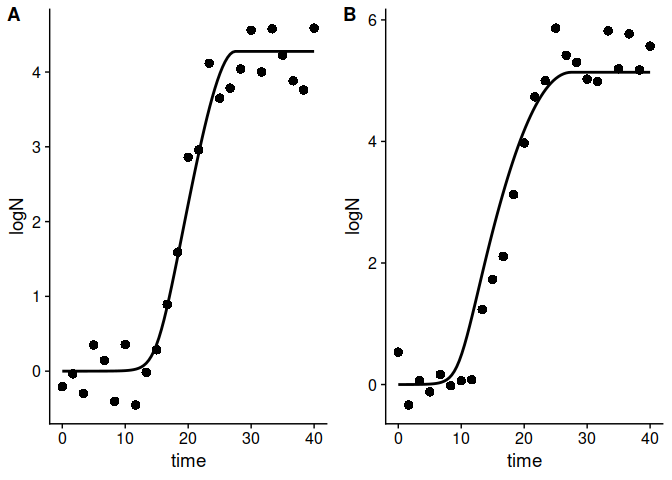This function generates an individual plot for each experiment. Any environmental factor can be included in the plot by passing the name of the factor to the add_factor argument. Note that the name must be identical to the one used for model definition.

plot(global_fit, add_factor = "temperature")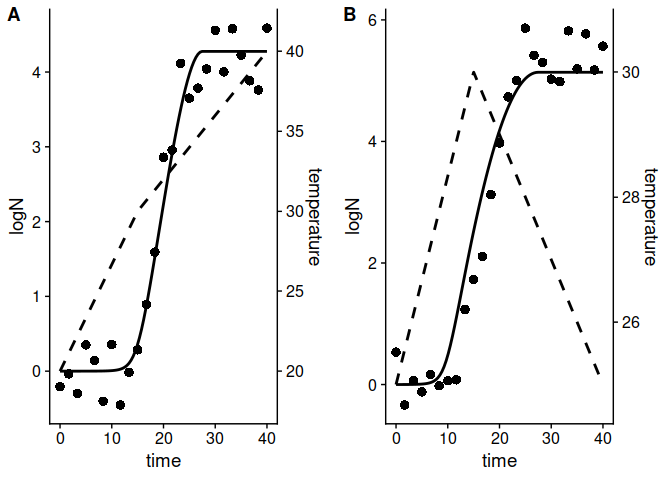The function includes additional arguments to edit several aesthetics of the plot. A full list of arguments can be found in the help page of plot.FitDynamicGrowth. The function returns an object of class ggplot, so it can be edited further using additional layers.

plot(global_fit, add_factor = "temperature",
label_x = "Storage time (h)",
label_y1 = "Size of the population (log CFU/g)",
label_y2 = "Temperature (ºC)",
line_col = "maroon", line_size = 2,
line_type2 = 1, line_col2 = "darkgrey"
)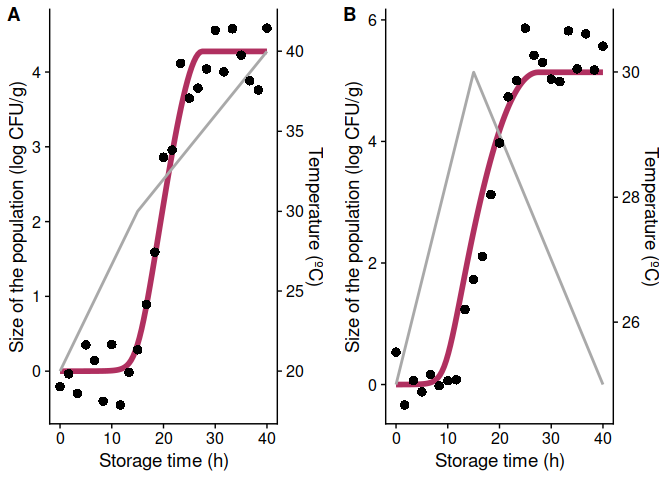## One-step fitting using MCMC algorithms

Numerical algorithms based on Markov Chains have been suggested as an alternative to non-linear regression for dynamic models. For that reason, biogrowth includes the function fit_MCMC_growth() that uses the interface included in the FME package to the Adaptive Monte Carlo algorithm by Haario et al. (2006). The arguments and the requirements of this function are identical to those of fit_dynamic_growth(). The only difference is that this function has the additional argument niter, which defines the number of samples from the Markov Chain. Hence, we will repeat the previous code to define the model parameters and the data.


data("example_dynamic_growth")
data("example_env_conditions")

sec_model_names <- c(temperature = "CPM",
aw= "CPM")

known_pars <- list(Nmax = 1e4,  # Primary model
N0 = 1e0, Q0 = 1e-3,  # Initial values of the primary model
mu_opt = 4, # mu_opt of the gamma model
temperature_n = 1, temperature_xmax = 40, # Secondary model for temperature
aw_xmax = 1, aw_xmin = .9, aw_n = 1  # Secondary model for water activity
)

my_start <- list(temperature_xmin = 25,
temperature_xopt = 35,
aw_xopt = .95)

Then, we can call the fit_MCMC_growth() using these arguments plus the argument niter that we will set to 100.

my_MCMC_fit <- fit_MCMC_growth(example_dynamic_growth, example_env_conditions,
my_start,
known_pars,
sec_model_names,
niter = 100)
#> number of accepted runs: 14 out of 100 (14%)

This function returns an instance of FitDynamicGrowthMCMC with 7 entries:

• fit_results: object returned by modMCMC().
• best_prediction: an instance of DynamicGrowth with the prediction corresponding to the fitted model.
• env_conditions: a tibble with the environmental conditions used for the simulations.
• data: data used to fit the model.
• starting: starting values used for parameter estimation.
• known: model parameters considered known.
• sec_models: type of secondary model for each environmental factor.

This class implements several S3 methods to aid in the the visualization of the results. A call to summary() returns the statistics of the Markov Chain.

summary(my_MCMC_fit)
#>      temperature_xmin temperature_xopt    aw_xopt
#> mean        26.067993        34.113044 0.92493536
#> sd           1.653552         2.326923 0.03863235
#> min         24.134938        28.885328 0.89152389
#> max         30.009495        38.058707 1.01043052
#> q025        24.710026        32.989925 0.89529239
#> q050        25.327366        33.748002 0.90238350
#> q075        27.204646        35.208507 0.96060785

Moreover, it includes methods for residuals, coef, vcov, deviance, fitted and predict. It also includes a plot method to compare the data against the fitted model.

plot(my_MCMC_fit)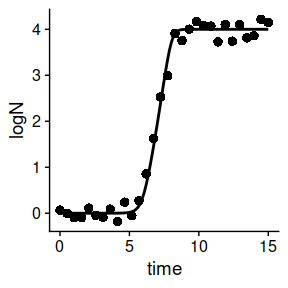As well as for fit_dynamic_growth(), the environmental conditions can be added to the plot using the add_factor argument. Other aesthetics can be edited passing additional arguments to plot (a full list can be found in the help page of plot.FitDynamicGrowthMCMC).

plot(my_MCMC_fit, add_factor = "temperature",
point_col = "steelblue", point_shape = 2, point_size = 6)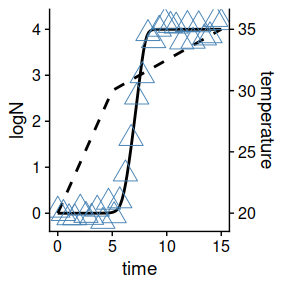## Global fitting using MCMC algorithms

Following the same logic as with the fit_MCMC_growth() function, fit_multiple_growth_MCMC() serves as an alternative to fit_multiple_growth() that uses an MCMC fitting algorithm instead of non-linear regression. The arguments of this function are identical to those of fit_multiple_growth() with the addition of niter, which defines the number of iterations from the MCMC sample.

Therefore, the definition of the data to use for the fit is identical.

data("multiple_experiments")

As well as the model definition.

## For each environmental factor, we need to defined a model

sec_names <- c(temperature = "CPM", pH = "CPM")

## Any model parameter can be fixed

known <- list(Nmax = 1e8, N0 = 1e0, Q0 = 1e-3,
temperature_n = 2, temperature_xmin = 20, temperature_xmax = 35,
pH_n = 2, pH_xmin = 5.5, pH_xmax = 7.5, pH_xopt = 6.5)

## The rest require starting values for model fitting

start <- list(mu_opt = .8, temperature_xopt = 30)

Then, the function can be called. Note that the MCMC algorithm is stochastic, so we will set the seed before fitting to grant reproducibility. Additionally, we will define upper and lower bounds for this function by passing the arguments lower and upper to modMCMC. For further ways to edit the fitting, please check the help page of modMCMC().

set.seed(12412)
global_MCMC <- fit_multiple_growth_MCMC(start, multiple_experiments, known, sec_names,
niter = 100,
lower = c(.2, 29),  # lower limits of the model parameters
upper = c(1.6, 34))  # upper limits of the model parameters
#> number of accepted runs: 14 out of 100 (14%)

This function returns a list of class FitMultipleDynamicGrowthMCMC with the same entries as FitMultipleDynamicGrowth. It also implements S3 methods to inspect the parameter estimates

summary(global_MCMC)
#>          mu_opt temperature_xopt
#> mean 0.54824071       30.3830315
#> sd   0.08199758        0.7297392
#> min  0.45625004       29.0379803
#> max  0.80000000       33.1855454
#> q025 0.47736567       29.6356637
#> q050 0.51539399       30.0434791
#> q075 0.57479388       31.1219113

Or to plot the predictions of the fitted model against the data.

plot(global_MCMC)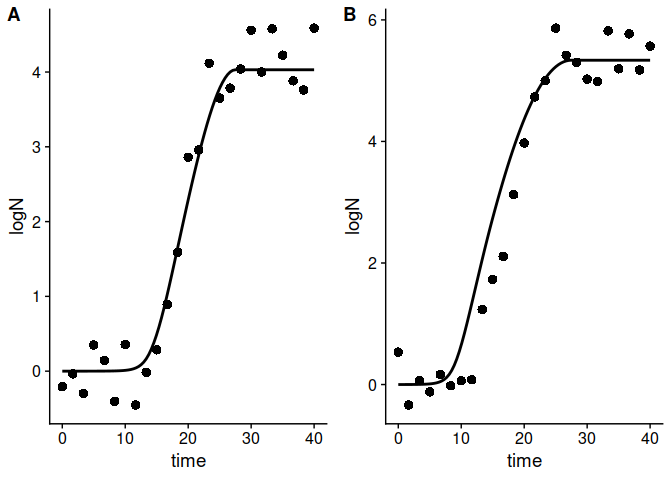Any environmental factor can be included in the plot using the add_factor argument. Also, the aesthetics of the plot can be edited passing additional arguments to plot (see the help page of plot.FitMultipleGrowthMCMC).

plot(global_MCMC, add_factor = "temperature",
line_col = "grey",
line_col2 = "blue", line_size2 = .5, line_type2 = 3)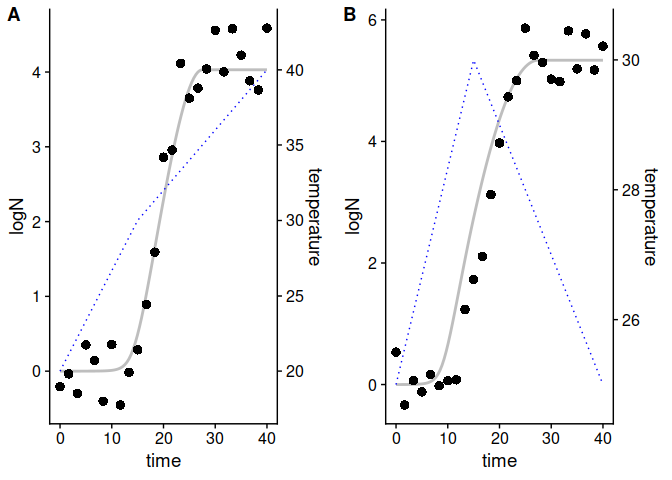## Stochastic prediction based on an MCMC fit

The function predict_MCMC_growth() makes stochastic predictions based on parameter distributions estimated using fit_MCMC_growth() or fit_multiple_growth_MCMC(). This function has 5 arguments:

• MCMCfit an instance of FitDynamicGrowthMCMC returned by fit_MCMC_growth(), or an instance of FitMultipleGrowthMCMC returned by fit_multiple_growth_MCMC().
• times vector of time points for the calculations.
• env_conditions tibble describing the (dynamic) environmental conditions.
• niter number of samples for the Monte Carlo calculations.
• newpars can be used to use different parameter values to those used for model fitting.

For this first example, we will use the same data we used previously to illustrate the use of the fit_MCMC_growth() function. The environmental conditions were defined by example_env_conditions

example_env_conditions
#> # A tibble: 3 × 3
#>    time temperature    aw
#>   <dbl>       <dbl> <dbl>
#> 1     0          20  0.99
#> 2     5          30  0.95
#> 3    15          35  0.9

This function estimates the credible intervals based on the quantiles of the predicted population size at each time point. Hence, their precision depends on the number of time points and the number of simulations. If the number of time points is too low, the prediction interval would not be “smooth”. On the other hand, if the number of simulations is too low, the credible interval would vary between repetitions of the same calculation.

As an example, we will use 5 time points uniformly distributed between 0 and 15

my_times <- seq(0, 15, length = 5)

and 100 iterations.

niter <- 100

Once we have defined every argument, we can call the predict_MCMC_growth() function.


my_MCMC_prediction <- predict_MCMC_growth(my_MCMC_fit,
my_times,
example_env_conditions,
niter)

This function returns an instance of MCMCgrowth with 5 entries:

• sample a tibble with the sample of model parameters used for the simulations.
• simulations a tibble with the results of every individual simulation used.
• quantiles a tibble providing the calculated quantiles (5th, 10th, 50th, 90th, 95th) of the population size for each time point.
• model the instance of FitDynamicGrowthMCMC used for the predictions.
• env_conditions a tibble with the environmental conditions of the simulations.

Hence, the quantiles at each time point can be retrieved from quantiles

my_MCMC_prediction\$quantiles
#> # A tibble: 5 × 7
#>    time      q50   q10      q90   q05     q95   m_logN
#>   <dbl>    <dbl> <dbl>    <dbl> <dbl>   <dbl>    <dbl>
#> 1  0    0         0    0         0    0       0
#> 2  3.75 0.000412  0    0.000689  0    0.00103 0.000445
#> 3  7.5  2.43      1.89 2.89      1.89 3.74    2.56
#> 4 11.2  4.00      4.00 4.00      3.98 4.00    4.00
#> 5 15    4         4.00 4         4.00 4       4.00

This class implements an S3 method for plot to visualize the prediction interval.

plot(my_MCMC_prediction)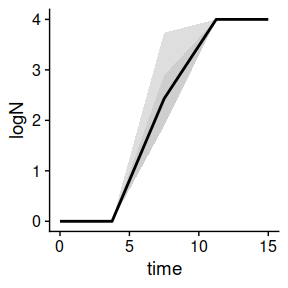In this plot, the solid line represents the median of the simulations, whereas the two shaded areas represent the space between the 10th and 90th, and 5th and 95th quantiles. As shown in this plot, the prediction interval is far from smooth. The reason for that is the low number of time points used for the calculations. Consequently, we will repeat them using 100 time points instead of 5:

better_prediction <- predict_MCMC_growth(my_MCMC_fit,
seq(0, 15, length = 100),
example_env_conditions,
niter)

If we visualize the new prediction interval

plot(better_prediction)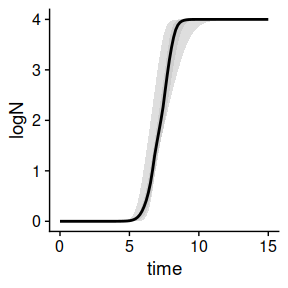it is now smoother. However, the prediction interval is still odd. Even if it is smooth, there are several inflection points that are hard to justify based on the model equations. They are the result of the low number of Monte Carlo samples used for the simulations. Hence, this number should be increased to obtain reliable intervals (not done in this vignette due to excessive compilation time).

By default, the predict_MCMC_growth function uses every parameter value estimated from the fit. This can be a limitation for simulations. For instance, the initial population size of a population of pathogenic species in an experiment is much higher than the one usually found in a food product. Also, it could be of interest to disregard the uncertainty of one parameter estimate. For that reason, the function includes the newpars argument, which can be used to assign a value (disregarding uncertainty) to one or more parameters. For instance, we could define an initial population size of 10, and a $$aw_{opt}$$ of 0.96.

other_prediction <- predict_MCMC_growth(my_MCMC_fit,
seq(0, 15, length = 100),
example_env_conditions,
niter,
newpars = list(aw_xopt = .96,
N0 = 10
)
)
plot(other_prediction)`